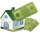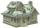# Exponential equation + interest, deposits and loan math - math problems

#### Number of problems found: 8

• Semiannually compound interestIf you deposit \$5000 into an account paying 8.25% annual interest compounded semiannually, how long until there is \$9350 in the account?
• Compound InterestIf you deposit \$6000 in an account paying 6.5% annual interest compounded quarterly, how long until there is \$12600 in the account?
• Mortage hypo loanThe Jonáš family decided to buy an older apartment, which cost EUR 30,000. They found EUR 17,000 and took the loan with the bank for the remaining amount. What interest did they receive if they repaid this amount for 15 years at EUR 120 per month?
• Investment1000\$ is invested at 10% compound interest. What factor is the capital multiplied by each year? How much will be there after n=12 years?
• Repay, interest, loanRamchacha takes a loan amount of 240000 from a bank for constructing a house at the rate of simple interest of 12% per annum. After 1 yr. of taking the loan he rents the house at the rate of 5200 per month. Determine the number of years he would take to r
• InterestWhat is the annual interest rate on your account if we put \$x and after \$n days received \$y?
• Compound interestCalculate time when the deposit in the bank with interest 2.5% p.a. doubles.
• When will I be a millionaire?Barry monthly send 280 euros to the bank, which he deposits bear interest of 2.1% p. A. Calculate how many months must Barry save to save 1000000 euros? Inflation, interest rate changes, or bank failures are ignored.

We apologize, but in this category are not a lot of examples.
Do you have an exciting math question or word problem that you can't solve? Ask a question or post a math problem, and we can try to solve it.

We will send a solution to your e-mail address. Solved examples are also published here. Please enter the e-mail correctly and check whether you don't have a full mailbox.

Exponential equation - math problems. Interest, deposits and loan math - math problems.